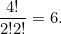# An almighty coincidence

December 2007

Imagine picking the four hymn numbers out of a hat. First note that four-hymn combinations with one 1-digit number and three 3-digit numbers come in four types: the one digit number can occur in first, second, third or last place of the selection. So the overall chance of picking such a combination is equal to:

 Chance of picking a combination with 1-digit number in 1st place + chance of picking a combination with 1-digit number in 2nd place + chance of picking a combination with 1-digit number in 3rd place + chance of picking a combination with 1-digit number in 4th place.

Each of the terms in this sum is equal to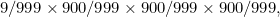so the overall chance of picking four hymn numbers such that one of them has 1 digit and the others have three digits is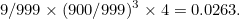Now for the chance of picking a combination with two 2-digit numbers and two 3-digit numbers. There are 6 ways in which to choose the positions of the two 2-digit numbers within the string of four numbers, so this time the selection comes in 6 different types:

•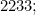•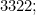•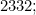•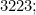•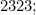•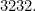The chance of picking a combination of each individual type is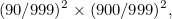so the overall chance is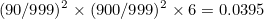document

In general, the number of ways you can choose a set of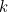positions within a sequence of length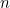is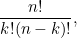where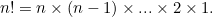In our examples, we first had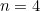with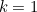, giving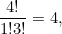and thenwith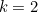, giving